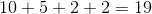# SSAT Middle Level Math : How to find the perimeter of a trapezoid

## Example Questions

← Previous 1

### Example Question #1 : Trapezoids

Find the perimeter of the trapezoid.

Note: Figure not drawn to scale.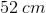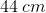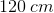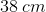Explanation:

To find the perimeter of a trapezoid, add the lengths of the sides: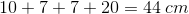### Example Question #2 : Trapezoids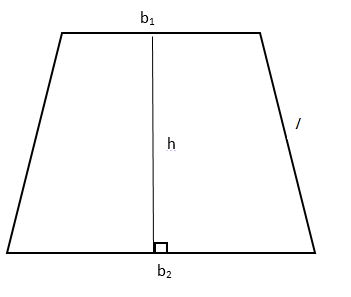Find the perimeter of the given trapezoid if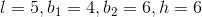19

20

18

14

20

Explanation:

To find the perimeter, we must find the sum of the OUTER edges: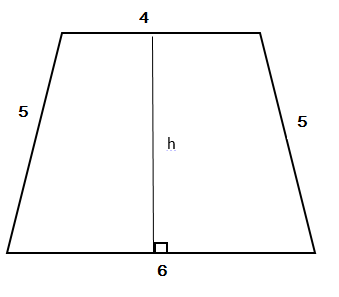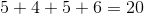Notice that the height wasn't included in our calculation.

### Example Question #3 : TrapezoidsFind the perimeter of the given trapezoid if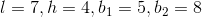24

31

20

27

27

Explanation:

In order to find the perimeter of the trapezoid, we must find the sum of the outer edges: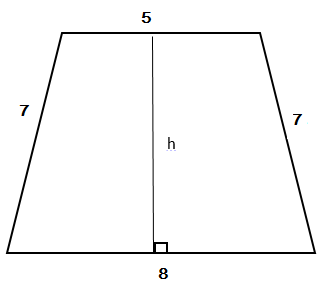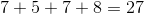Notice that we didn't use height in our calculation.

### Example Question #4 : TrapezoidsFind the perimeter of the given trapezoid if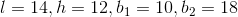54

22

44

56

56

Explanation:

To find the perimeter, we need to find the sum of the outer edges: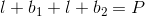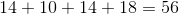Notice that we didn't use height in our calculation.

### Example Question #1 : Trapezoids

Measured in units, the bases of a trapezoid areand, the lengths are, and the height is unknown.  What is the perimeter of the trapezoid in units?Impossible to calculate.Explanation:

The perimeter is solved by adding the two bases together,and, along with both the lengths,.

Therefore the equation becomes,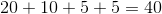### Example Question #6 : Trapezoids

Measured in units, the bases of a trapezoid areand, the lengths are, and the height is unknown.  What is the perimeter of the trapezoid in units?Impossible to calculate.Explanation:

The perimeter is solved by adding the two bases together,and, along with both lengths,.

Therefore the equation becomes,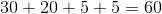### Example Question #7 : Trapezoids

Measured in units, the bases of a trapezoid areand, the lengths are, and the height is unknown.  What is the perimeter of the trapezoid in units?Impossible to calculate.Explanation:

The perimeter is solved by adding the two bases together,and, along with both lengths,.

Therefore the equation becomes,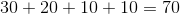### Example Question #8 : Trapezoids

Measured in units, the bases of a trapezoid areand, the lengths are, and the height is unknown.  What is the perimeter of the trapezoid in units?Impossible to calculate.Explanation:

The perimeter is solved by adding the two bases together,and, along with both the lengths,.

Therefore the equation becomes,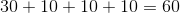### Example Question #9 : Trapezoids

Measured in units, the bases of a trapezoid areand, the lengths are, and the height is unknown.  What is the perimeter of the trapezoid in units?Impossible to calculate.Explanation:

The perimeter is solved by adding the two bases together,and, along with both the lengths,.

Therefore the equation becomes,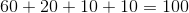### Example Question #10 : Trapezoids

Measured in units, the bases of a trapezoid areand, the lengths are, and the height is unknown.  What is the perimeter of the trapezoid in units?

Impossible to calculate.The perimeter is solved by adding the two bases together,and, along with both the lengths,.